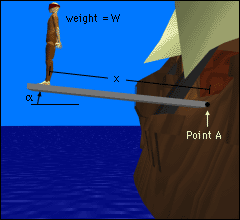Ch 4. Moments/Equivalent Systems Multimedia Engineering Statics Moment2-D Scalar Moment3-D Scalar Moment3-D Vector Couples and Equiv. System Distributed Loads, Intro
 Chapter 1. Basics 2. Vectors 3. Forces 4. Moments 5. Rigid Bodies 6. Structures 7. Centroids/Inertia 8. Internal Loads 9. Friction 10. Work & Energy Appendix Basic Math Units Sections Search eBooks Dynamics Fluids Math Mechanics Statics Thermodynamics Author(s): Kurt Gramoll ©Kurt GramollSTATICS - CASE STUDY IntroductionProblem Diagram After attempting to form a workers' union with his shipmates, a seaman is forced to walk the plank for mutiny. The moment generated at the base of the plank depends on the man's weight, his location, and the angle of the boat. What is known: The man has a weight of W lb and is standing a distance of x ft from the base of the plank. The boat is tilted an angle of α, measured in degrees. Question What is the moment generated about point A, which is located at the base of the plank? Approach Determine the perpendicular distance from point A to the line of action of the force as a function of x and α. Calculate the moment about point A by multiplying the force times the perpendicular distance.

Practice Homework and Test problems now available in the 'Eng Statics' mobile app
Includes over 500 problems with complete detailed solutions.
Available now at the Google Play Store and Apple App Store.